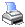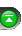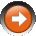﻿ ToTime()
 NinjaScript > Language Reference > Data > ToTime()Definition
Calculates an integer value representing time. NinjaScript uses the .NET DateTime structure which can be complicated for novice programmers. If you are familiar with C# you can directly use DateTime structure properties and methods for date and time comparisons otherwise use this method and the ToDay() method.

Integer representation of time is in the following format Hmmss where 7:30 AM would be 73000 and 2:15:12 PM would be 141512.

Method Return Value

An int value that represents the time.

Syntax
ToTime(DateTime time)

Parameters

 time A DateTime structure such as Time - See Time property

Examples

 // Only trade between 7:45 AM and 1:45 PM if (ToTime(Time) >= 74500 && ToTime(Time) <= 134500) {     // Strategy logic goes here }